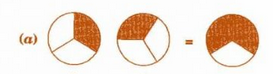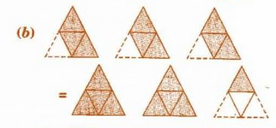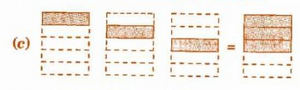Q

# Some pictures (a) to (c) are given below. Tell which of them show: 3 into 1/5 = 3/5, 2 into 1/3 = 2/3, 3 into 3/4= 2 1/4

2.  Some pictures (a) to (c) are given below. Tell which of them show:Views

As in option (c), In the Left-hand side, there are three figures in which the one part out of three-part is shaded and in the right-hand side, three out of five portions are shaded.

Hence this represents

.

Option (a) represents the equation pictorially.

Option (b) represents this equation pictorially.

Exams
Articles
Questions